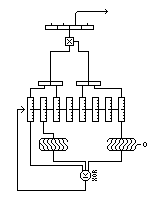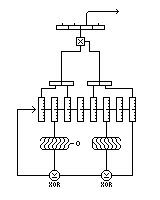[Next] [Up] [Previous] [Index]

# Other Stream Ciphers

The mixed congruential pseudorandom number generator, usually used in software, is one of the basic techniques of producing apparently random bits that we will examine here. This is the technique used to produce the numbers given by the RND() function in most dialects of BASIC. Modulo a constant, replace x by a times x plus b, where a and b are both constants. If a and b are large enough, the behavior of x, particularly its most significant bits, will seem random.

For the maximum period, which is the same as the modulus, for the mixed congruential generator which is:

```x' = ax + b (modulo m)
```

the conditions are:

• b must be relatively prime to the modulus
• a must be equal to 1 modulo every prime factor of the modulus
• a must be equal to 1 modulo 4 if 4 divides the modulus

as given in Seminumerical Algorithms(Knuth) and Random Number Generators(Janssen). Thus, for a modulus that is a power of 2, a must be equal to 1 modulo 4; for a modulus that is a power of 10, a must be equal to 1 modulo 20.

The most common method used for strengthening a mixed congruential generator is to use it as part of a MacLaren-Marsaglia random number generator.

Let us suppose that random binary bits are desired. Then, one uses one generator modulo two to some power, so that one is starting with numbers with a uniform distribution. Since the most significant bits of the output from such a generator have the longest period, one might take only the 4 or 8 most significant bits of the output.

A buffer, perhaps with 37 entries, containing 37 bytes or nibbles produced by that generator is used. Each time some bits are to be produced, a second mixed-congruential generator, operating modulo 37^n for some n, is used to pick one element from that buffer, which is used as the output of the full MacLaren-Marsaglia generator. Then the other mixed-congruential generator is used to supply a replacement value for the buffer element used.

Again, a simple MacLaren-Marsaglia generator is still not secure, although the paper in which one was cracked used one where all the bits of the binary MC generator were used and none were discarded. If only the first few bits are used, and a long binary MC generator, perhaps one requiring multi-precision arithmetic, is used, there is already a greater level of security present. But more elaborate constructs are again possible.

But there are many other techniques that can be applied to bytes or words, rather than bits, to produce a keystream to XOR with plaintext.

Gifford's cipher used only eight bytes of internal state, but produced a cipher that was only shown to have weaknesses after some very involved analysis.

It actually was a kind of shift-register cipher, but with the shifting being done by byte. The shift register was eight bytes long. Feedback involved taking three bytes from the register, and obtaining the new byte by XORing together one of the bytes, the arithmetic right shift of another byte, and the logical left shift of the third.

The output from the generator is produced by taking four bytes from the register, forming two 16-bit integers from them, and taking the second least-significant byte of their product. This output is what is XORed to the plaintext to produce ciphertext.

This diagram illustrates Gifford's cipher:As it is based on a nonlinear shift register, there is no way to ensure maximum period, but one problem with it is shrinkage of the state space, because a bit is discarded from the oldest shift register entry that is reused. That is easily corrected: for example, by a design like this:A stream cipher is any cipher which, like Vigenere, or that produced by a rotor machine, changes how it behaves during a message. Thus, most block cipher modes, other than Electronic Codebook Mode, produce stream ciphers.

A stream cipher which does produce pseudorandom bits to XOR with plaintext can be improved merely by substituting new values for the bytes of the plaintext from a secret table, both before and after the XOR.

Another way of using the output of a pseudorandom bit generator was developed by Terry Ritter, which he called Dynamic Substitution.

The principle is very simple. A secret table, with a random sequence of the 256 possible byte values, is used.

A message byte is replaced by its substitute in that table in order to encrypt it.

Then, a byte from the pseudorandom bit generator is taken. The two table entries corresponding to that byte, and the plaintext message byte, are swapped. In the event both the plaintext byte and the psudorandom byte are the same, nothing is done.

This is a simple, but secure technique. Every time a table entry is used, it is relocated somewhere else at random. So, since each table entry is used once and once only, no useful information about the table seems to be made available.

If one knows some corresponding plaintext and ciphertext, it is true that since you know that the table entry you encountered when one byte was enciphered was sent to the byte the PRNG sent it to at that time, and may stay there for a while, if that same byte turns up shortly after, you can conclude that the PRNG byte in the past is the same as the plaintext byte when the byte value turned up again.

However, one cannot expect a simple method of applying a keystream to plaintext to be perfect; this small weakness doesn't contradict the fact that this is a great improvement over simply XORing the keystream to the plaintext.

The main reason this technique may not become popular even after its patent expires is because it is an autokey method; the encipherment of plaintext bytes depends in part on the values of previous plaintext bytes. This is not good for error-propagation, which need not be a consideration (since once text is encrypted, it can be sent along with extensive error-correction; and encrypted texts are often compressed, which already results in wide propagation of any errors) but it is usually considered to be a problem.

The idea of shuffling elements in a table of the 256 different byte values can also be used to generate pseudorandom bytes.

One very popular stream cipher has been alleged to function as follows:

Using two variables that store one byte each, in addition to the table, generate bytes as follows:

Each iteration proceeds in this way:

• Increment A (modulo 256).
• Add the A-th element of the table to B (modulo 256).
• Use as the output byte the element of the table specified by the modulo-256 sum of the A-th element and the B-th element of the table.
• Exchange the A-th element and the B-th element of the table with each other.

The initial arrangement of the 256-byte table is created by a procedure involving a second 256-byte table. The table to be used in generating pseudorandom bytes is initialized to the numbers from 0 to 255 in order. The other table is filled with the bytes of the key repeated over and over until it is full.

Start again with A and B equal to zero.

Repeat the following steps 256 times:

• Replace B with the sum of B and the A-th element of both tables.
• Increment A.
• Swap the A-th element and the B-th element of the table to be used later, leaving the one containing the key alone.

In addition to the mixed congruential generator, where

```x(n+1)=a*x(n)+b
```

and, as noted, modulo 2^n, the condition for maximum period is that b must be odd, and a must be equal to 1 modulo 4, it is possible to use a quadratic congruential generator, of the form:

```x(n+1)=a*x(n)^2+b*x(n)+c
```

since this is not linear, it is harder to crack, and in fact one stream cipher bit source, using squaring as the function to move from one state to the next, is believed to be highly secure (the Blum-Blum-Shub cipher) - but this cipher uses a very large modulus, and therefore arithmetic on very large numbers, in the same fashion as RSA.

I had been informed, by one Vladimir Anashin, of the Russian State University for the Humanities, that the condition for maximum period for a quadratic congruential generator modulo 2^n is that the coefficients be the same, modulo 8, as a generator that gives the full period of 8 modulo 8. A computer search for satisfactory coefficients gave me the following 24 possible sets to choose from:

``` 1)  2  3  1
0  1  6  3  4  5  2  7

2) 2  3  3
0  3  6  5  4  7  2  1

3) 2  3  5
0  5  6  7  4  1  2  3

4) 2  3  7
0  7  6  1  4  3  2  5

5) 2  7  1
0  1  2  7  4  5  6  3

6) 2  7  3
0  3  2  1  4  7  6  5

7) 2  7  5
0  5  2  3  4  1  6  7

8) 2  7  7
0  7  2  5  4  3  6  1

9) 4  1  1
0  1  6  7  4  5  2  3

10) 4  1  3
0  3  2  5  4  7  6  1

11) 4  1  5
0  5  6  3  4  1  2  7

12) 4  1  7
0  7  2  1  4  3  6  5

13) 4  5  1
0  1  2  3  4  5  6  7

14) 4  5  3
0  3  6  1  4  7  2  5

15) 4  5  5
0  5  2  7  4  1  6  3

16) 4  5  7
0  7  6  5  4  3  2  1

17) 6  3  1
0  1  2  7  4  5  6  3

18) 6  3  3
0  3  2  1  4  7  6  5

19) 6  3  5
0  5  2  3  4  1  6  7

20) 6  3  7
0  7  2  5  4  3  6  1

21) 6  7  1
0  1  6  3  4  5  2  7

22) 6  7  3
0  3  6  5  4  7  2  1

23) 6  7  5
0  5  6  7  4  1  2  3

24) 6  7  7
0  7  6  1  4  3  2  5
```

The conditions for the quadratic congruential generator to have maximum period for any base are given in Seminumerical Algorithms, the second volume of Donald E. Knuth's The Art of Computer Programming in an exercise.

Where the recurrence is:

```        2
x' = a x  + b x + c (modulo m)
```

the conditions on a, b, and c required for the maximum period (which matches the modulus) are:

• c must be relatively prime to the modulus,
• b is equal to 1 modulo every odd prime factor of the modulus;
• a is equal to 0 modulo every odd prime factor of the modulus;
• if the modulus is divisible by 4,
• b is equal to 1 modulo 2, and
• a is equal to b-1 modulo 4;
• if the modulus is divisible by 2, either a is even and b is odd, which is the only possibility if the modulus is divisible also by 4, or a is odd and b is even;
• if the modulus is divisible by 9, either a is equal to 0 modulo 9, or
• b is equal to 1 modulo 9, and
• a times c is equal to 6 modulo 9.

Another important pseudorandom number generator is the Mersenne Twister, which is described on the preceding page as it is a form of linear-feedback shift register.

One other stream cipher of interest is given its own section.

[Next] [Up] [Previous] [Index]